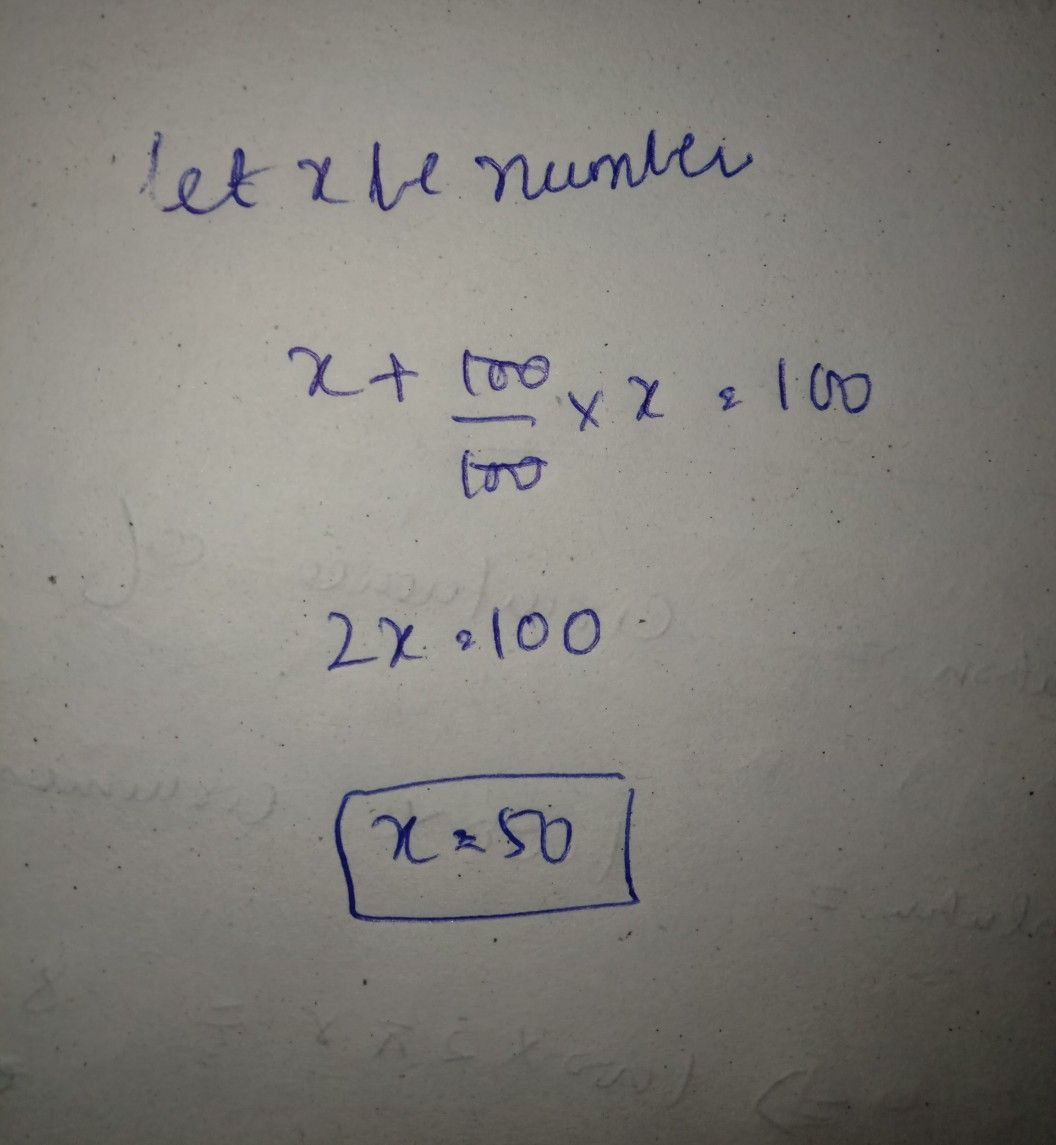Symbol
Problemwhen $0$ $1n\left(9uad$ $|$ $by100A_{0}$ becomes $e$ $e$ $0$